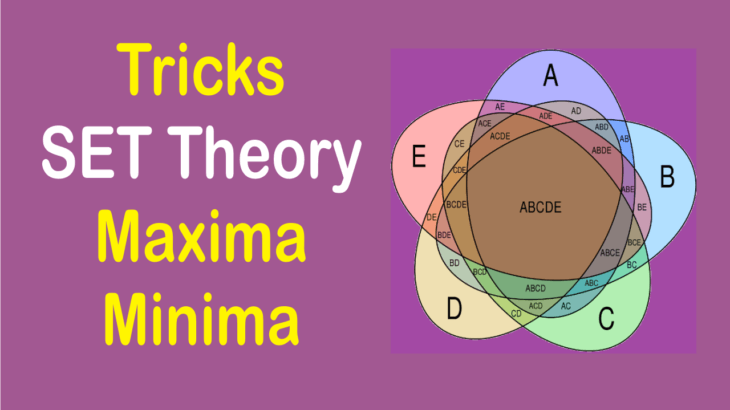# Maxima Minima Concept of Set Theory and Venn Diagram

CAT 2020 MOCK Test Series at INR 1000/- only.

Maxima Minima Concept based on Set theory or Venn diagram is very important for CAT exam not only in Quant section but more so in LRDI section. Many varieties of questions can be framed from this area. In most of the cases, we are required to find maximum and minimum value in Venn diagram related questions.

Here is the video to understand the concept and trick to solve questions based on maxima-minima.
The problems can further be categorized into two cases:
1. When the Union of the sets is NOT fixed
2. When the Union of the set is fixed.
In this video, we have covered both the cases and with the help of examples, we will understand the application of the concept on Maxima Minima.

For Online CAT Quant course click the below link:

1.Ayush says: